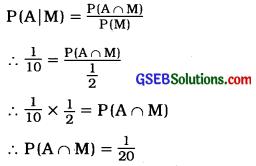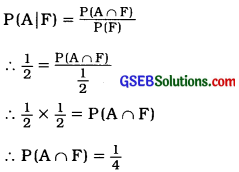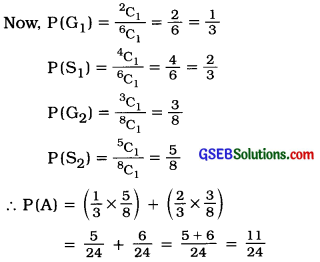# GSEB Solutions Class 12 Statistics Part 2 Chapter 1 Probability Ex 1.4

Gujarat Board Statistics Class 12 GSEB Solutions Part 2 Chapter 1 Probability Ex 1.4 Textbook Exercise Questions and Answers.

## Gujarat Board Textbook Solutions Class 12 Statistics Part 2 Chapter 1 Probability Ex 1.4

Question 1.
There are two children in a family. If the first child is a girl then find the probability that both the children in the family are girls.
Let, B = Boy and G = Girl.
∴ The sample space for the family having two children is expressed as follows:
U = {BB, BG, GB, GG}
∴ n = 4
A = Event that first child is a girl
= {GB, GG}
∴ Favourable outcomes for the event A is m = 2.
∴ P(A) = $$\frac{m}{n}$$ = $$\frac{2}{4}$$ = $$\frac{1}{2}$$
B = Event that both the children are girls = {GG}
∴ Favourable outcome for the event B is m = 1.
P(B) = $$\frac{m}{n}$$ = $$\frac{1}{4}$$
A ∩ B = Event that both children are girls Event B
∴ P(A ∩ B) = P(B) = $$\frac{1}{4}$$
Now, B|A = Conditional event that first child is a girl, then second child is also a girl.
∴ P(B|A) = $$\frac{P(A \cap B)}{P(A)}$$
= $$\frac{\frac{1}{4}}{\frac{1}{2}}$$ = $$\frac{1}{4} \times \frac{2}{1}$$ = $$\frac{1}{2}$$Question 2.
Two six-faced balanced dice are thrown simultaneously. If the sum of numbers on both the dice is more than 7 then find the probability that both the dice show same numbers.
Two six-faced balanced dice are thrown simultaneously.
∴ Total number of primary outcomes n = 62
= 36
A = Event that the sum of numbers on both the dice is more than 7, i.e., 8, 9, 10, 11 or 12
= {(2, 6), (3, 5), (4, 4), (5, 3), (6, 2), (3, 6), (4, 5), (5, 4), (6, 3), (4, 6), (5, 5), (6, 4), (5, 6), (6, 5), (6, 6)}
∴ Favourable outcomes for the event A is m = 15.
∴ p(A) = $$\frac{m}{n}$$ = $$\frac{15}{36}$$
B = Event that both the dice show same numbers
= {(1, 1), (2, 2), (3, 3), (4, 4), (5, 5), (6, 6)}
∴ Favourable outcomes. for the event B is m = 6.
A ∩ B = Event that the sum of numbers on both the dice is more than 7 and both the dice show same numbers
= {(4, 4), (5, 5), (6, 6)}
∴ Favourable outcomes for the event A ∩ B is m = 3.
∴ P(A ∩ B) = $$\frac{m}{n}$$ = $$\frac{3}{36}$$
Now, B | A = Conditional event B knowing that the sum of numbers on both the dice is more than 7.
According to law of conditional Probability
p(B|A) = $$\frac{P(A \cap B)}{P(A)}$$
= $$\frac{\frac{3}{36}}{\frac{15}{36}}$$ = $$\frac{3}{36} \times \frac{36}{15}$$ = $$\frac{1}{5}$$

Question 3.
Among the various vehicle owners visiting a petrol pump, 80% vehicle owners visit to fill petrol in their vehicle and 60% vehicle owners visit to fill air in their vehicles. 50 % vehicle owners visit to fill air and petrol in their vehicle. Find the probability for the following events:
(1) If a vehicle owner has come to fill petrol in his vehicle, then that vehicle owner will fill air in his vehicle.
(2) If a vehicle owner has come to fill air in his vehicle, then that vehicle owner will fill petrol in his vehicle.
A = Event that vehicle owners fill petrol in their vehicles
∴ P(A) = $$\frac{80}{100}$$ = $$\frac{4}{5}$$
B = Event that vehicle owners fill air in their vehicles
∴ P(B) = $$\frac{60}{100}$$ = $$\frac{3}{5}$$
A ∩ B = Event that vehicle owners fill both petrol and air in their vehicles
∴ P(A ∩ B) = $$\frac{50}{100}$$ = $$\frac{1}{2}$$(1) B|A = Conditional event B knowing that vehicle owners visited the petrol pump to fill the petrol in their vehicles
According to law of conditional probability,
P(B|A) = $$\frac{P(A \cap B)}{P(A)}$$
= $$\frac{\frac{1}{2}}{\frac{4}{5}}$$ = $$\frac{1}{2} \times \frac{5}{4}$$ = $$\frac{5}{8}$$

(2) A | B = Conditional event A knowing that vehicle owners visited the petrol pump to fill air in their vehicle.
According to law of conditional probability,
P(A|B) = $$\frac{P(A \cap B)}{P(A)}$$
= $$\frac{\frac{1}{2}}{\frac{3}{5}}$$ = $$\frac{1}{2} \times \frac{5}{3}$$ = $$\frac{5}{6}$$

Question 4.
80 % customers hold saving account and 50% customers hold current account of a nationalised bank. 90% of the customers hold at least one of the saving account and the current account. If one of the account holders randomly selected from this bank holds a current account, find the probability that he holds a saving account.
A = Event that the customers hold the saving account
∴ P(A) = $$\frac{80}{100}$$ = $$\frac{4}{5}$$
B = Event that the customers hold the current account
∴ P(B) = $$\frac{50}{100}$$ = $$\frac{1}{2}$$
A ∪ B = Event that the customers hold at least one of the saving account and the current account
∴ P(A ∪ B) = $$\frac{90}{100}$$ = $$\frac{9}{10}$$
A ∩ B = Event that the customers hold both the saving and current accounts
Now, P(A ∪ B) = P(A) + P(B) – P(A ∩ B)
∴ $$\frac{9}{10}$$ = $$\frac{4}{5}+\frac{1}{2}$$ – P(A ∩ B)
∴ P(A ∩ B) = 
= $$\frac{8+5-9}{10}$$ = $$\frac{4}{10}$$ = $$\frac{2}{5}$$
A|B = Conditional event A knowing that the customers hold current account.
According to law of conditional probability.
p(B|A) = $$\frac{P(A \cap B)}{P(A)}$$
= $$\frac{\frac{2}{5}}{\frac{1}{2}}$$ = $$\frac{2}{5} \times \frac{2}{1}$$ = $$\frac{4}{5}$$

Question 5.
If P(A) = $$\frac{2}{3}$$, P(B) = $$\frac{3}{5}$$ and P(B|A) = $$\frac{3}{4}$$ for two events in the sample space of a random experiment, then find P(A|B).
Here, P(A) = $$\frac{2}{3}$$, P(B) = $$\frac{3}{5}$$ and P(B|A) = $$\frac{3}{4}$$ are given.
p(B|A) = $$\frac{P(A \cap B)}{P(A)}$$
∴ P(A ∩ B) = P(B|A) – P(A)
= $$\frac{3}{4} \times \frac{2}{3}$$ = $$\frac{1}{2}$$
Now, P(A|B) = $$\frac{P(A \cap B)}{P(A)}$$
= $$\frac{\frac{1}{2}}{\frac{3}{5}}$$ = $$\frac{1}{2} \times \frac{5}{3}$$ = $$\frac{5}{6}$$Question 6.
If P (M) = P (F) = $$\frac{1}{2}$$, P (A | M) = $$\frac{1}{10}$$ and P(A|F) = $$\frac{1}{2}$$ for events A, M and F, then find P(A ∩ M) and P (A ∩ F).
Here, P(M) = P(F) = $$\frac{1}{2}$$
P(M) = $$\frac{1}{2}$$ and P(F) = $$\frac{1}{2}$$ are given.

P(A ∩ M):P(A ∩ F):Question 7.
There are 2 gold coins and 4 silver coins in a box. The other box contains 3 gold and 5 silver coins. One coin is selected from each box. Find the probability that one of the selected coins is a gold coin and the other is a silver coin.
In a box there are total (2 gold + 4 silver) 6 coins.
In the other box there are total (3 gold + 5 silver) 8 coins.
A = Event that a coin selected from each box is a gold coin and a silver coin.
There are two options of occuring the event A:
→ A gold coin (G1) is selected from the first box and a silver coin (S2) is selected from the other box.
OR
→ A silver coin (S1) is selected from the first box and a gold coin (G2) is selected from the other box.
∴ A = (G1 ∩ S2) ∪ (S1 ∩ G2)
∴ P(A) = (G1 ∩ S2) + (S1 ∩ G2)
(∵ (G1 ∩ S2) and (S1 ∩ G2) are mutually exclusive events.)
= P(G1) ∙ P(S2) + P(S1) ∙ P(G2)
(∵ G1, S2, S1, G2 are independent events.) ………. (1)Question 8.
One joint family has 3 sons and 2 daughters whereas the other joint family has 2 sons and 4 daughters. One joint family is selected from two joint families and a child is randomly selected from that family. Find the probability that the selected child is a girl.
In the first joint family there are total (3 sons + 2 daughters) 5 children.
In the second joint family there are total (2 sons + 4 daughters) 6 children.
Suppose, event F1 = First family is selected and ,
event F2 = Second family is selected
∴ P(F1) = P(F2) = $$\frac{1}{2}$$
A = Event that the child is a girl.
Event A can occur in two different ways :
→ First family F1 selected and child is a girl.

OR

→ Second family selected and child is a girl.
∴ A = (F1 ∩ G1) u (F2 ∩ G2)
According to the law of multiplication of probability,
P(A) = [P(G1|F1) ∙ P(F1)] + [P(G2|F2) ∙ P(F2)] …………… (1)
Now, P(G1|F1) = Conditional probability of the event that the selected child is a girls knowing that the first family is selected
= $$\frac{{ }^{2} C_{1}}{{ }^{5} C_{1}}$$ = $$\frac{2}{5}$$

P(G2|F2) = Conditional probability of the event that the selected child is a girl knowing that the
second family is selected.
= $$\frac{{ }^{4} \mathrm{C}_{1}}{{ }^{6} \mathrm{C}_{1}}$$ = $$\frac{4}{6}$$ = $$\frac{2}{3}$$
Putting the values in the result (1),
P(A) = $$\left(\frac{2}{5} \times \frac{1}{2}\right)$$ + $$\left(\frac{2}{3} \times \frac{1}{2}\right)$$
= $$\frac{1}{5}+\frac{1}{3}$$ = $$\frac{3+5}{15}$$ = $$\frac{8}{15}$$Question 9.
There are 10 ice cream cones in a box of which 3 cones weigh less than the specification and the rest of the 7 cones have the specified weight. Two cones are randomly selected one by one with replacement. Find the probability that both the cones selected weigh less than the specified weight.
There are 10 ice cream cones in a box of which 3 cones weigh less than the specified weight and 7 cones have the specified weight. T\vo cones are randomly selected one by one with replacement.
∴ Total primary outcomes n = 10C1 = 10
A = Event that the cone selected in the first trial weigh less than the specified weight.
∴ Favourable outcomes for the event A is m = 3C1 = 3.
∴ P(A) = $$\frac{m}{n}$$ = $$\frac{3}{10}$$
Cone is selected with replacement. So in the second trial also n = 10C1 = 10
B = Event that the cone selected in second trial weigh less than the specified weight
∴ Favourable outcomes for the event B is m = 3C1 = 3
∴ P(B) = $$\frac{m}{n}$$ = $$\frac{3}{10}$$
Now, A ∩ B = Event that both the cones selected weigh less than the specified weight.
Events A and B are independent events.
∴ P(A ∩ B) = P(A) ∙ P(B)
= $$\frac{3}{10} \times \frac{3}{10}$$
= $$\frac{9}{100}$$

Question 10.
There are 10 CDs in a CD rack in which 6 are action film CDs and 4 are drama film CDs. Two CDs are randomly selected one by one without replacement from this box. Find the probability that the first selected CD is of action film and the second CD is of drama film.
There are total (6 action film + 4 drama film) 10 CDs in a CD rack.
One CD is selected from the CD rack.
∴ Total primary outcomes is n = 10C1 = 10
A = Event that the CD selected in the first trial is of action film
∴ Favourable outcomes for the event A is
m = 6C1 = 6.
∴ p(A) = $$\frac{m}{n}$$ = $$\frac{6}{10}$$
Now, two CDs are randomly selected one by one without replacement. So there will be 9 CDs of which 5 are action film CDs and 4 are drama film CDs.
∴ In the second trial total primary outcomes is n = 9C1 = 9.
Now, B|A = Event that second CD selected is of drama film knowing that the first CD selected is of action film
∴ Favourable outcomes for the event B|A is
m = 4C1 =4.
∴ P(B|A) = $$\frac{m}{n}$$ = $$\frac{4}{9}$$
AnB = Event that the first selected CD is of action film and the second CD is of drama film
According to law of multiplication of probability,
P(A ∩ B) = P(B|A) ∙ P(A)
= $$\frac{4}{9} \times \frac{6}{10}$$ = $$\frac{4}{15}$$Question 11.
If two balanced dice are thrown, then find the probability that
(1) at least one die shows number 5
(2) the first die shows the number 5 or 6 and the other die shows an even number.
A die is thrown.
∴ U = {1, 2, 3, 4, 5, 6} ∴ n = 6

(1) A = Event that the first die shows number 5
∴ P(A) = $$\frac{1}{6}$$
B = Event that the second die shows number 5
∴ P(B) = $$\frac{1}{6}$$
A ∩ B = Event that both dices show number 5
∴ P(A ∩ B) = P(A) ∙ (B) = $$\frac{1}{6} \times \frac{1}{6}$$ = $$\frac{1}{36}$$
Now, A ∪ B = Event that at least one die shows number 5
∴ P(A ∪ B) = P(A) + P(B) – P(A ∩ B)
= $$\frac{1}{6}+\frac{1}{6}-\frac{1}{36}$$
= $$\frac{6+6-1}{36}$$
= $$\frac{11}{36}$$

(2) A = Event that the first die shows the number 5 or 6
B = Event that the second die shows an even number = {2, 4, 6}
∴ P(A) = $$\frac{2}{6}$$ = $$\frac{1}{3}$$
B = Event that the second die shows an even number
= {2, 4, 6}
∴ P(B) = $$\frac{3}{6}$$ = $$\frac{1}{2}$$
Now, A ∩ B = Event that the first die shows the number 5 or 6 and the second die shows an even number.
∴ P(A ∩ B) = P(A) ∙ P(B) (∵ A and B are independent events)
= $$\frac{1}{3} \times \frac{1}{2}$$
= $$\frac{1}{6}$$Question 12.
A problem in Mathematics is given to Tania, Kathan and Kirti to solve. The probabilities of them solving the problem correctly are $$\frac{2}{3}$$, $$\frac{3}{4}$$ and $$\frac{1}{2}$$ respectively. Find the probability that the problem is solved correctly.
A = Event that Tania solves the problem correctly
∴ P(A) = $$\frac{2}{3}$$
∴ P(A’) = 1 – P(A)
= 1 – $$\frac{2}{3}$$ = $$\frac{1}{3}$$

B = Event that Kathan solves the problem correctly
∴ P(B) = $$\frac{3}{4}$$
∴ P(B’) = 1 – P(B)
= 1 – $$\frac{3}{4}$$ = $$\frac{1}{4}$$

C = Event that Kirti solves the problem correctly
∴ P(C) = $$\frac{1}{2}$$
∴ P(C’) = 1 – P(C)
= 1 – $$\frac{1}{2}$$ = $$\frac{1}{2}$$

Now, A’ ∩ B’ ∩ C’ = Event that the problem is not solved
∴ P(A’ ∩ B ‘ ∩ C’) = P(A’) × P(B’) × P(C’)
= $$\frac{1}{3} \times \frac{1}{4} \times \frac{1}{2}$$
= $$\frac{1}{24}$$

Hence, the probability that the problem is solved correctly,
= 1 – P(A’ ∩ B’ ∩ C’)
= 1 – $$\frac{1}{24}$$ = $$\frac{23}{24}$$Question 13.
Person A can hit the target in 3 out of 5 attempts whereas person B can hit the target in 5 out of 6 attempts. If both of them attempt simultaneously, find the probability that the target is hit.
A = Event that person A can hit the target
∴ P (A) = $$\frac{3}{5}$$
B = Event that person B can hit the target
∴ P (B) = $$\frac{5}{6}$$
A ∩ B = Event that persons A and B can hit the target
P(A ∩ B) = P(A) ∙ P(B) = $$\frac{3}{5} \times \frac{5}{6}$$ = $$\frac{1}{2}$$
(∵ A and B can hit the target independently.)
Now, A ∪ B = Event that the target is hit.
According to law of addition of probability,
P(A ∪ B) = P(A) + P(B) – P(A ∩ B)
= $$\frac{3}{5}+\frac{5}{6}-\frac{1}{2}$$
= $$\frac{18+25-15}{30}$$
= $$\frac{28}{30}$$
= $$\frac{14}{15}$$

Question 14.
Person A speaks truth in 90 % cases whereas person B speaks truth in 80 % cases. Find the probability that persons A and B differ in stating the same fact.
A = Event that A speaks truth
∴ P(A) = $$\frac{90}{100}$$ = 0.9
∴ P(A ) = 1 – P(A)
= 1 – 0.9
= 0.1

B = Event that B speaks truth
∴ P(B) = $$\frac{80}{100}$$ = 0.9
∴ P(B) = 1 – P(B)
= 1 – 0.8
= 0.2

There are two different ways in which persons A and B differ in stating the same fact:
→ A speaks truth and B does not speak truth, i.e., event A ∩ B’
OR
→ A does not speak truth and B speaks truth, i.e., event A ∩ B
Events A and B are independent events. So A’ and B’ are also independent events.
Hence, the probability that persons A and B differ in stating the same fact
= P(A ∩ B’) u P(A’ ∩ B)
= [P(A) ∙ P(B’)] + [P(A’) ∙ P(B)J
= [0.9 × 0.2] + [0.1 × 0.8]
= 0.18 + 0.08
= 0.26Question 15.
If three events A, B and C of a random experiment are independent events and P(A) = 0.2, P(B) = 0.3 and P(C) = 0.5, then find P(A ∪ B ∪ C).
Here, P(A) = 0.2, P(B) = 0.3 and P(C) = 0.5 are given.
A, B and C are independent events.
∴ P(A ∩ B) = P(A) ∙ P(B)
= 0.2 × 0.3
= 0.06

P(A ∩ C) = P(A) ∙ P(C)
= 0.2 × 0.5
= 0.1

P(B ∩ C) = P(B) ∙ P(C)
= 0.3 × 0.5 = 0.15

P(A ∩ B ∩ C) = P(A) ∙ P(B) ∙ P(C)
= 0.2 × 0.3 × 0.5
= 0.03

Now, P(A ∪ B ∪ C)
= P(A) + P(B) + P (C) – P (A ∩ B) – P (A ∩ C) -P(B ∩ C) + P(A ∩ B ∩ C)
= 0.2 + 0.3 + 0.5 – 0.06 – 0.1 – 0.15 + 0.03 = 0.72
= 0.1